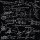1. Circle and squareAn ABCD square with a side length of 100 mm is given. Calculate the radius of the circle that passes through the vertices B, C and the center of the side AD.
2. Magnified cubeIf the lengths of the edges of the cube are extended by 5 cm, its volume will increase by 485 cm3. Determine the surface of both the original and the magnified cube.
3. BirthdaysIn the classroom, students always give candy to their classmates on their birthdays. The birthday person always gives each one candy, and he does not give himself. A total of 650 candies were distributed in the class per year. How many students are in the
4. An equilateralAn equilateral triangle is inscribed in a square of side 1 unit long so that it has one common vertex with the square. What is the area of the inscribed triangle?
5. TV competitionIn the competition, 10 contestants answer five questions, one question per round. Anyone who answers correctly will receive as many points as the number of competitors answered incorrectly in that round. One of the contestants after the contest said: We
6. Conical bottleWhen a conical bottle rests on its flat base, the water in the bottle is 8 cm from it vertex. When the same conical bottle is turned upside down, the water level is 2 cm from its base. What is the height of the bottle?
7. The productThe product of a number plus that number and its inverse is two and one-half. What is the inverse of this number
8. FlowerbedWe enlarge the circular flower bed, so its radius increased by 3 m. The substrate consumption per enlarged flower bed was (at the same layer height as before magnification) nine times greater than before. Determine the original flowerbed radius.
9. A rectangle 2A rectangle has a diagonal length of 74cm. Its side lengths are in ratio 5:3. Find its side lengths.
10. Square sideIf we enlarge the square side a = 5m, its area will increase by 10,25%. How many percent will the side of the square increase? How many percent will it increase the circumference of the square?
11. Sides of right angled triangleOne leg is 1 m shorter than the hypotenuse, and the second leg is 2 m shorter than the hypotenuse. Find the lengths of all sides of the right-angled triangle.
12. Before yesterdayHe merchant adds a sale sign in his shop window to the showed pair of shoes in the morning: "Today by p% cheaper than yesterday. " After a while, however, he decided that the sign saying: "Today 62.5% cheaper than the day before yesterday". Determine the
13. Medians in right triangleIt is given a right triangle, angle C is 90 degrees. I know it medians t1 = 8 cm and median t2 = 12 cm. .. How to calculate the length of the sides?
14. Faces diagonalsIf the diagonals of a cuboid are x, y, and z (wall diagonals or three faces) respectively than find the volume of a cuboid. Solve for x=1.3, y=1, z=1.2
15. Two chordsCalculate the length of chord AB and perpendicular chord BC to circle if AB is 4 cm from the center of the circle and BC 8 cm from the center of the circle.
16. Geometric progressiobIf the sum of four consective terms of geometric progression is 80 and arithmetic mean of second and fourth term is 30 then find terms?
17. Working aloneTom and Chandri are doing household chores. Chandri can do the work twice as fast as Tom. If they work together, they can finish the work in 5 hours. How long does it take Tom working alone to do the same work?
18. Ratio of sidesCalculate the area of a circle that has the same circumference as the circumference of the rectangle inscribed with a circle with a radius of r 9 cm so that its sides are in ratio 2 to 7.
19. RT sidesFind the sides of a rectangular triangle if legs a + b = 17cm and the radius of the written circle ρ = 2cm.
20. Perimeter of RTFind the circumference of the rectangular triangle if the sum of its legs is 22.5 cm and its area is 62.5 cm2.

Do you have an interesting mathematical word problem that you can't solve it? Submit math problem, and we can try to solve it.

We will send a solution to your e-mail address. Solved examples are also published here. Please enter the e-mail correctly and check whether you don't have a full mailbox.

Please do not submit problems from current active competitions such as Mathematical Olympiad, correspondence seminars etc...

Looking for help with calculating roots of a quadratic equation?